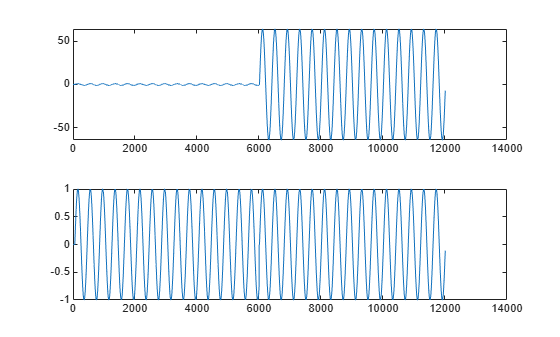# gain

Gain of CIC filter System object

## Syntax

``g = gain(sysobj)``
``g = gain(sysobj,j)``

## Description

example

````g = gain(sysobj)` returns the gain of the CIC filter System object™.When `sysobj` is a `dsp.CICDecimator` object, the `gain` function returns the gain for the overall CIC decimator.When `sysobj` is a `dsp.CICInterpolator` object, the `gain` function returns the gain of the 2Nth stage of the CIC interpolation filter, where N is the number of filter sections. For more details, see `g`.```
````g = gain(sysobj,j)` returns the gain of the `j`th section of a CIC interpolation filter. When you omit `j`, the function assumes that `j` is 2N, and returns the gain of the last section of the filter. This syntax does not apply when `sysobj` is a `dsp.CICDecimator` object.```

## Examples

collapse all

To compare the performance of two interpolators, one a CIC filter and the other an FIR filter, use the `gain` function to adjust the CIC filter output amplitude to match the FIR filter output amplitude.

Start by creating an input data set, a sinusoidal signal x.

```fs = 1000; % Input sampling frequency. t = 0:1/fs:1.5; % Signal length = 1501 samples. x = sin(2*pi*10*t); % Amplitude = 1 sinusoid. x = x';```

Design a cascade of two `dsp.FIRInterpolator `objects with an overall interpolation factor of 4.

```l = 4; % Interpolation factor for FIR filter. d = fdesign.interpolator(l); firInterp = design(d,'multistage','SystemObject',true);```

Run the data through the interpolator.

`yfir = firInterp(x);`

Design a `dsp.CICInterpolator` object with an interpolation factor set 4, differential delay set to 1, and the number of sections set to 4.

```r = 4; % Interpolation factor for the CIC filter. d = fdesign.interpolator(r,'cic'); cicInterp = design(d,'multisection','SystemObject',true);```

Run the same data through the filter.

`ycic = cicInterp(x);`

Use the `gain` function to adjust the CIC filter output amplitude to match the FIR filter output amplitude.

```gaincic = gain(cicInterp); subplot(211); plot([yfir; double(ycic)]); subplot(212) plot([yfir; double(ycic)/gain(cicInterp)]);```After correcting for the gain induced by the CIC interpolator, in the second subplot you can see that the FIR filter and the CIC filter provide nearly identical interpolation.

This gain equals the gain of the last section of the CIC filter. To confirm, correct the FIR filter amplitude using `gain`($\mathrm{cicInterp}$,$2\mathit{N}$). If $\mathit{N}$ is the number of integrator and comb sections of the CIC filter, then $2\mathit{N}$ is the last section of the CIC filter. $\mathit{N}$ is given by cicInterp.NumSections.

The second subplot shows that the FIR filter and CIC filter provide nearly identical interpolation when the correction gain equals the gain of the last section of the CIC filter.

```subplot(212); plot([yfir; double(ycic)/gain(cicInterp,2*cicInterp.NumSections)]);```## Input Arguments

collapse all

Input CIC filter, specified as one of the following filter System objects:

Index of the CIC interpolator stage for which the gain is computed, specified as a positive scalar.

Data Types: `single` | `double`

## Output Arguments

collapse all

Gain of the CIC filter, returned a scalar. When the input `sysobj` is:

• `dsp.CICDecimator` –– The `gain` function returns the gain for the overall CIC decimator.

• `dsp.CICInterpolator` –– The CIC interpolator inserts zeros into the input data stream, reducing the filter overall gain by 1/R, where R is the interpolation factor, to account for the added zero-valued samples. Therefore, the gain of a CIC interpolator is (RM)N/R, where N is the number of filter sections and M is the filter differential delay. The `gain` function returns this value.

Data Types: `single` | `double`

Introduced in R2011a

## SupportGet trial now